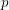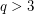# 3 is a primitive root modulo primes of the form 16 q^4 + 1, where q>3 is prime

 Importance: Medium ✭✭
 Author(s):
 Subject: Number Theory
 Keywords:
 Recomm. for undergrads: no
 Posted by: princeps on: February 25th, 2012
Conjectureis a primitive root modulofor all primes, whereis prime.

## Bibliography

* indicates original appearance(s) of problem.

### group theory answer

Using group theory, the multiplicative group of order p=16q^4+1 has order p-1=16q^4. Using lagrange's theorem, the order of any element divides the order of the group. Therefore, any element is either a primitive root, a quadratic residue, or a qth power residue mod 16q^4+1. Using the laws of quadratic reciprocity, 3 is a quadratic residue modulo a prime if and only if the prime is congruent to plus or minus 1 mod 12. Since q>3 is a prime and therefore not divisible by 3, 16q^4=1(mod 3), so 16q^4+1=2(mod 3). That means that 16q^4+1=5(mod 12), and therefore 3 is not a quadratic residue mod p. Therefore the only thing left to prove is that 3 is not a qth power residue.

## Comment viewing options

Select your preferred way to display the comments and click "Save settings" to activate your changes.